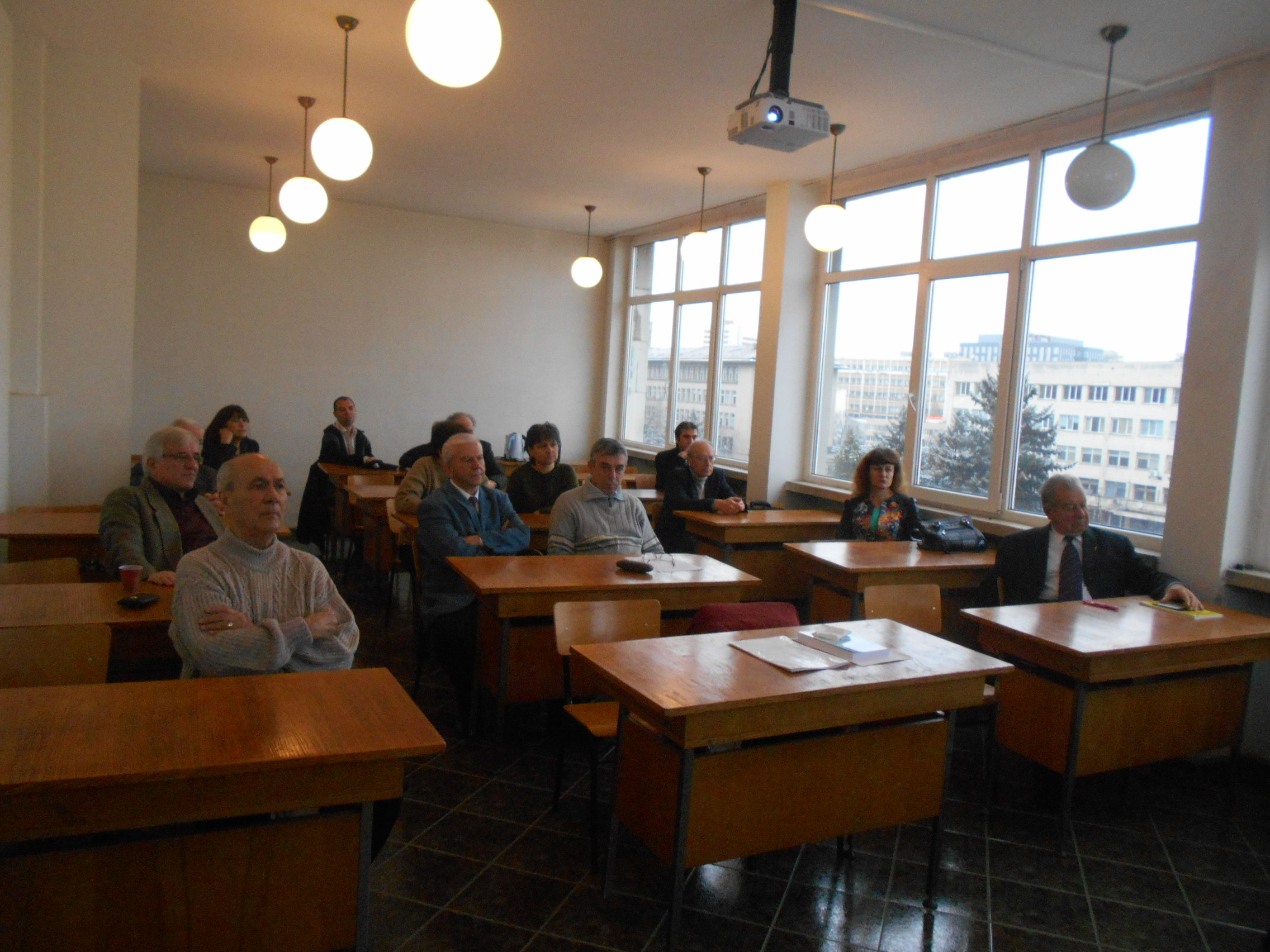﻿ Analysis, Geometry and Topology Department

### Analysis, Geometry and Topology Department

Academic Staff Photos Joint Seminar

Ivan Dimovski at 85

### Historical remarks

The Department of Analysis, Geometry and Topology (AGT) was created in March 2011 by merging the former departments Complex Analysis, Geometry and Topology, and Real and Functional Analysis..

The Department of Complex Analysis was one of the first departments within the Institute of Mathematics and Informatics at the Bulgarian Academy of Sciences with clearly identified research areas and well-developed scientific potential. It was one of the departments originating from the former Department of Advanced Analysis, directed by Academician Lyubomir Tchakalov until 1962. In 1962 it was divided into three separate departments: Complex Analysis, Real and Functional Analysis, and Differential Equations. Previous chairs of the department of Complex Analysis have been Academician Lyubomir Iliev (1962–1988), and Corresponding Member Ivan Dimovski (1988–2004). Since 2004 chair of the department is Corresponding Member Oleg Mushkarov. Initially, research in the department was focused on topics from traditional areas of classical function theory like geometric function theory, distribution of zeroes of entire and meromorphic functions. Gradually, modern areas like multi-dimensional complex analysis, complex geometry, special functions, integral transforms, fractional and operational calculi were added. In the course of time, three main directions of scientific research were formed, which by the year 2011 were grouped into the following thematic projects: functions of a complex variable, transform methods and special functions, several complex variables, and complex geometry.

The Department of Geometry and Topology was established in 1993 by merging the former Department of Geometry and Department of Topology. Until March 2011 the department was headed by Assoc. Prof. Georgi Ganchev. The Department of Geometry was one of the first departments at the Institute of Mathematics. Throughout the years chairs of the department have been Academician B. Petkanchin and Prof. G. Stanilov. The Department of Topology was founded in 1971 and has been headed by Prof. D. Doichinov and Prof. S. Nedev. The main areas of research in the Department of Geometry and Topology have been differential geometry of manifolds and general topology.

The Department of Real and Functional Analysis was founded in 1958 and has been headed by Prof. Y. Tagamlitzki, Prof. S. Troyansky, and Prof. K. Kirtchev. The main areasof research in the department have been the geometry of Banach spaces and spectral theory of linear and non-linear operators.

Since its formation in 2011 until 2020 the Department of Analysis, Geometry and Topology has been headed by Corresponding Member Oleg Mushkarov. At present, research in the department has been concentrated in two basic research projects with the participation of 23 scientists.

From July 2020 head of the department is Corresponding Member Nikolai Nikolov.

### Several Complex Variables, Differential Geometry and Topology

Investigations in the theory of holomorphic functions of several complex variables and the geometry of complex and almost complex manifolds. Investigations in the field of differential geometry of Riemannian manifolds, almost Hermitian manifolds and almost contact metric manifolds, differential geometry of Riemannian and Kaehlerian manifolds with a distribution. Investigations in the field of topology and tomography related to convex geometry and infinite dimensional topology. Education and supervision of MSc and PhD students in the fields of the research project.

Main fields of research:

• Invariant (pseudo-) metrics on domains in n-dimensional complex space.
• Geometric and analytic properties and characteristics of complex convex domains.
• Geometry of four-dimensional manifolds.
• Complex surfaces endowed with additional geometric structures.
• Hermitian geometry of twistor spaces of four-dimensional Riemannian manifolds.
• Contact geometry of twistor spaces of odd-dimensional Riemannian manifolds.
• Differential geometry of surfaces and hypersurfaces in Euclidean and Minkowski spaces.
• Local theory of surfaces in pseudo-Euclidean spaces with neutral metric.
• Convex projections of sets in Banach spaces and infinite dimesional topology.

Research team:

Corresponding Member DSc Nikolai Nikolov, Corresponding Member DSc Oleg Mushkarov, Corresponding Member DSc Stefan Ivanov, Prof. DSc Johann Davidov, Prof. PhD Velichka Milousheva (Coordinator of the Project), Prof. PhD Ludmil Katzarkov, Assoc. Prof. PhD Vestislav Apostolov, Assist. Prof. PhD Petar Petrov, Assist. Prof. PhD Stoyu Barov, Assist. Prof. Strashimir Popvasilev, Mathematician Antoni Rangachev, Viktoria Bencheva (PhD Student), Marin Genov (PhD Student).

### Transform Methods, Special Functions and Complex Analysis

Research studies in the areas of applied mathematical analysis in real and complex domains, and functional analysis; publication of surveys, scientific articles and educational materials; publishing of a specialized international journal: Fractional Calculus and Applied Analysis; organizing specialized international conferences on the subject as the traditional events: Transform Methods and Special Functions, Complex Analysis and Applications, Geomеtric Function Theory and Applications, etc.; publishing proceedings of these conferences and selected works of renowned Bulgarian mathematicians; education and supervision of MSc and PhD students in these areas.

Main fields of research:

• Integral transforms and transmutation operators.
• Special functions, entire functions, orthogonal polynomials.
• Fractional calculus (integration and differentiation of fractional order): theory, generalizations, applications to mathematical models of systems of fractional order.
• Operational and convolutional calculi of local and nonlocal boundary value problems for differential operators of integer and fractional order, generalization of the Duhamel principle for space variables in problems of mathematical physics.
• Geometric theory of functions of one complex variable: investigation of special classes of univalent functions and relationships between them, coefficients estimates, distortion theorems.
• Distribution and geometry of zeros of classes of entire functions and polynomials.
• Approximation theory by rational functions in the complex plane: Pade approximations, best rational approximations in Chebyshev and Lp-metrics, rational Chebyshev approximations of real-valued functions, measure theory and orthogonal polynomials.
• Spectral theory of self-adjoint operators, Laplacians and quantum Hamiltonians, and applications.

Research team:

Academician DSc Stanimir Troyanski, Corresponding Member DSc Ivan Dimovski, Prof. DSc Peter Rusev, Prof. DSc Jordanka Paneva-Konovska (Coordinator of the Project), Prof. DSc Virginia Kiryakova, Prof. DSc Ralitza Kovacheva, Prof. DSc Stepan Terzian, Assoc. Prof. PhD Emilia Bazhlekova, Assist. Prof. PhD Nikolay Ikonomov.